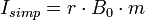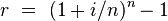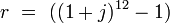APR and EAR are both annual interest rate which is used for calculation of annual interest on a loan. Full form of APR is annual percentage rate and EAR is effective annual percentage rate. But there are following differences between APR and EAR.

1. Calculation Method

APR is calculated by using simple interest rate formula. For this, we need total interest payment, principal amount and time in years. But for calculating EAR, we need to calculate compound interest rate. After this, we also need to add different fees in calculated compound interest rate.

2. Cost of Borrowing

Because in APR, we do not add different fees, so, it is just nominal cost of borrowing which we have to pay in simple annual interest after calculation of APR. In EAR, we have to pay service charges, late fee and origination fees.

3. Example

Example of APR

A bank issued loan of $100 for 7 months with simple annual interest of 15%. This simple annual interest rate will be APR. Example of EAR A bank issued loan of$ 100 for 6 months at 5%  per month plus $10 fees per month. If we convert 5% per month and$ 10 fees per month into annual interest rate, it will be annual percentage rate.

4. Formula of Calculation of APR and EAR

Basic Formula of calculation of APRBasic Formula of calculation of EARThe effective interest rate is calculated as if compounded annually. The effective rate is calculated in the following way, where r is the effective annual rate, i the nominal rate, and n the number of compounding periods per year (for example, 12 for monthly compounding):If the monthly interest rate j is known and remains constant throughout the year, the effective annual rate can be calculated as follows:Wikipedia References : APR and EAR.

## $type=three$a=hide$cm=hide$s=hide$show=/2019/06/10-steps-to-become-better-investor.html$l=hide

Name

false
ltr
item
Accounting Education: APR Vs EAR
APR Vs EAR